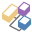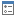#Numerics

## Overview

NumericValues represent all the different values of the measure chips used in the drop zones used to draw the visualization for a given data point. In Custom Visuals, each data point includes a composite of multiple values used to show different graphical capabilities (like the text value, color value, size value etc.).

• From version: 2020.20

## Properties

###additionalValues

The datapoint's numeric values as defined in the "AdditionalValues" drop zone. If no value chip was added to the drop zone it returns an empty array;

``````const datapoint = cvApi2.resultSet.data.getCurrentTrellisData().datapoints;

``````

###color

color: Value[]

The datapoint's numeric values as defined in the "Color" drop zone. If no value chip was added to the drop zone it returns an empty array;

``````const datapoint = cvApi2.resultSet.data.getCurrentTrellisData().datapoints;

console.log("current Color numeric value is : " + datapoint.numerics.color.rawValue);
``````

###indicator

indicator: Value[]

The datapoint's numeric values as defined in the "Indicator" drop zone. If no value chip was added to the drop zone it returns an empty array;

``````const datapoint = cvApi2.resultSet.data.getCurrentTrellisData().datapoints;

console.log("current Indicator numeric value is : " + datapoint.numerics.indicator.rawValue);
``````

###labels

labels: Value[]

The datapoint's numeric values as defined in the "Labels" drop zone. If no value chip was added to the drop zone it returns an empty array;

``````const datapoint = cvApi2.resultSet.data.getCurrentTrellisData().datapoints;

console.log("current labels numeric value is : " + datapoint.numerics.labels.rawValue);
``````

###shape

shape: Value[]

The datapoint's numeric values as defined in the "Shape" drop zone. If no value chip was added to the drop zone it returns an empty array;

``````const datapoint = cvApi2.resultSet.data.getCurrentTrellisData().datapoints;

console.log("current Shape numeric value is : " + datapoint.numerics.shape.rawValue);
``````

###size

size: Value[]

The datapoint's numeric values as defined in the "Size" drop zone. If no value chip was added to the drop zone it returns an empty array;

``````const datapoint = cvApi2.resultSet.data.getCurrentTrellisData().datapoints;

console.log("current Size numeric value is : " + datapoint.numerics.size.rawValue);
``````

###tooltip

tooltip: Value[]

The datapoint's numeric values as defined in the "Tooltip" drop zone. If no value chip was added to the drop zone it returns an empty array;

``````const datapoint = cvApi2.resultSet.data.getCurrentTrellisData().datapoints;

console.log("current Tooltip numeric value is : " + datapoint.numerics.tooltip.rawValue);
``````

###value

value: Value

The datapoint's numeric value as defined in the "Values" drop zone. If no value chip was added to the drop zone it returns undefined;

``````const datapoint = cvApi2.resultSet.data.getCurrentTrellisData().datapoints;

console.log("current Valuenumeric value is : " + datapoint.numerics.value.rawValue);
``````

###xAxis

xAxis: Value

The datapoint's numeric value as defined in the "XAxis" drop zone. If no value chip was added to the drop zone it returns undefined;

``````const datapoint = cvApi2.resultSet.data.getCurrentTrellisData().datapoints;

console.log("current XAxis numeric value is : " + datapoint.numerics.xAxis.rawValue);
``````

###yAxis

yAxis: Value

The datapoint's numeric value as defined in the "YAxis" drop zone. If no value chip was added to the drop zone it returns undefined;

``````const datapoint = cvApi2.resultSet.data.getCurrentTrellisData().datapoints;

console.log("current XAxis numeric value is : " + datapoint.numerics.yAxis.rawValue);
``````